# 深度学习笔记

d2l 深度学习笔记，如有错误，欢迎拍砖GitHub项目地址：AI-Project

### 〇、技术路线图

flowchart TD
A[softmax 回归] -->|无法拟合 XOR 函数| B[多层感知机]
B --> |高像素图片作为输入，模型参数爆炸| C[卷积]
C -->|数据的长宽下降太快| D[填充]
C -->|数据的长宽下降太慢| E[步幅]
C -->|缓解卷积对位置敏感| F[池化]
C -->|多模式识别与组合| G[多通道输入/输出]



### 一、softmax 回归

• 回归估计是一个连续值
• 分类预测是一个离散类别

#### 2. 分类应用举例

• MINIST
• ImageNet
• human-protein-atlas-image-classification (Kaggle)
• malware-classification (Kaggle)
• jigsaw-comment-classification (Kaggle)

#### 3. 从回归到多类分类 – 均方损失

$y = [y_1, y_2, , ... , y_n]^T$

$y_i=\left\{\begin{array}{l}1 \text { if } i=y \\ 0 \text { otherwise }\end{array}\right.$

$\hat{y}=\underset{i}{\operatorname{argmax}} o_i$

#### 4. 无校验比例

$O_y - O_i \geq \Delta (y, i)$

#### 5. 校验比例

$\hat{y_i} = \frac{\exp(O_i)}{\sum_{k} \exp(O_k)}$

#### 6. 交叉熵损失

$l(\mathbf{y}, \hat{\mathbf{y}})=-\sum_i y_i \log \hat{y}_i=-\log \hat{y}_y$

PS：因为除了 $i = y$ 的情况，$i$ 为其他值时 $y_i$ 为 0

$\partial_{o_i} l(\mathbf{y}, \hat{\mathbf{y}})=\operatorname{softmax}(\mathbf{o})_i-y_i$

#### 7. 总结

• Softmax回归是一个多类分类模型
• 使用Softmax操作子得到每个类的预测置信度
• 使用交叉熵来衡量预测和标号的区别

### 二、多层感知机

#### 1. 什么是感知机

$o = \sigma \left(\left<W, X\right> + b\right)$

$\sigma(x) = \left\{\begin{array}{l}1 \text { if } x > 0 \\ -1 \text { otherwise }\end{array}\right.$

#### 2. 训练感知机

1. 初始化：w = 0, b = 0
2. 如果分类分错：$y_i [\left< W, X_i \right>] \leq 0 \text{ then } w \gets w + y_i x_i \text{ and } b \gets b + y_i$
3. 停止条件：所有类别都分对的时候停止

$l\left(y, X, W\right) = max\left(0, -y\left<W, X\right>\right)$

#### 3. 收敛定理

• 数据在半径 $r$
• 余量 $\rho$ 将数据点分两类
• $\lvert\lvert w \rvert\rvert^{2} + b^2 \leq 1$

#### 4. XOR问题 (Minsky & Papert, 1969)

        |
1   |   -1
|
-----------------
|
-1   |    1
|


#### 5. 多层感知机

    　   |
①    |    ②
|
-------------------
|
③    |    ④
|

• 先学一次：对于竖线，左边为 $+$ ，右边为 $-$
• 再学一次：对于横线：上边为 $+$ ，下边为 $-$#### 6. 单隐藏层

• 输入：$\mathbf{x} \in \mathbb{R}^{n}$
• 隐藏层：$\mathbf{W}_1 \in \mathbb{R}^{m \times n}, \mathbf{b}_1 \in \mathbb{R}^m$
• 输出层：$\mathbf{w}_2 \in \mathbb{R}^{m}, \mathbf{b}_2 \in \mathbb{R}$

$~~~~~~~~~\mathbf{h} = \sigma \left( \mathbf{W}_1 + \mathbf{b}_1 \right)$

$~~~~~~~~~\mathbf{o} = \mathbf{w}_2^\mathbf{T} \mathbf{h} + \mathbf{b}_2$

$\sigma$ 是按元素的激活函数

Note: 为什么需要非线性的激活函数？

$\mathbf{hence} \space \mathbf{o} = \mathbf{w}_2^T \mathbf{W}_1 \mathbf{o} + b^{\prime}$ 依然是线性，折腾半天发现我们得到的依旧是一个最简单的线性模型。

#### 7. Sigmoid 激活函数

$sigmoid(x) = \frac{1}{1 + \mathbf{exp}(-x)}$#### 8. Tanh 激活函数

$tanh(x) = \frac{1 - \mathbf{exp}(-2x)}{1 + \mathbf{exp}(-2x)}$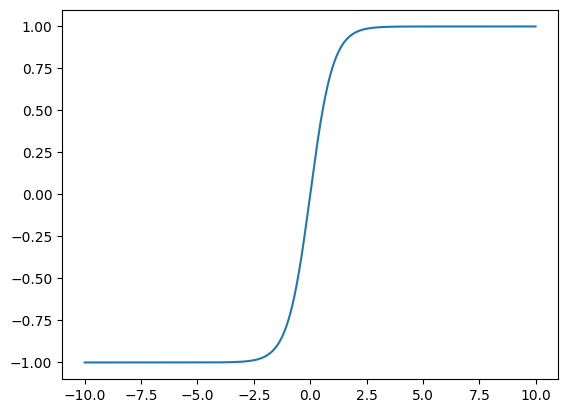#### 9. ReLU 激活函数

ReLU: rectified linear unit

$ReLU(x) = \mathbf{max}(x, 0)$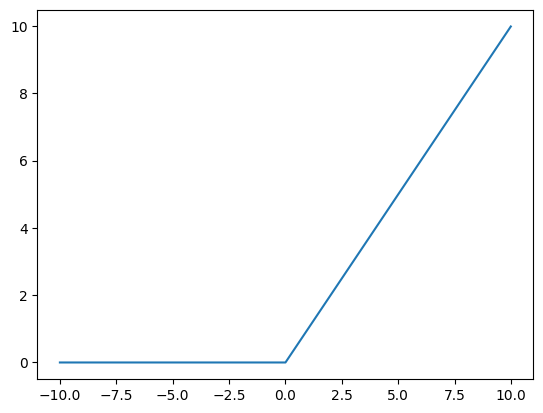#### 10. 多类分类：借鉴 softmax

$softmax$ 就是输出一个堆概率 $y_1, y_2, ..., y_k$，所有这些加起来等于1

$y_1, y_2, ..., y_k = softmax(o_1, o_1, ... , o_k)$

#### 11. 多隐藏层

$\mathbf{h}_1 = \sigma(\mathbf{W}_1\mathbf{x} + b_1)$

$\mathbf{h}_2 = \sigma(\mathbf{W}_2\mathbf{h}_1 + b_2)$

$\mathbf{h}_3 = \sigma(\mathbf{W}_3\mathbf{h}_2 + b_3)$

$\mathbf{o} = \mathbf{W}_4\mathbf{h}_3 + b_4$

• 隐藏层数
• 每层隐藏层的大小

#### 12. 总结

• 多层感知机使用隐藏层和激活函数来得到非线性模型
• 常用激活函数是Sigmoid, Tanh, ReLU
• 使用Softmax来处理多类分类
• 超参数为隐藏层数，和各个隐藏层大小

### 三、卷积层

#### 1. 识别器的原则

• 平移不变性（在图片的哪个位置都能识别到）
• 局部性（不需要看整张图就能识别）

#### 2. 重新考察全连接层

• 将输入和输出变形为矩阵（宽度，高度）
• 将权重变形为4-D张量 $(h, w)$$(h^\prime, w^\prime)$ $h_{i,j} = \sum_{k,l} w_{i,j,k,l}x_{k,l} = \sum_{a,b} v_{i,j,a,b}x_{i+a,j+b}$
• $v$$w$ 的重新索引 $v_{i,j,i+a,j+b}$

#### 3. 原则 #1 - 平移不变性

• x的平移导致h的平移 $h_{i,j} = \sum_{a,b} v_{i,j,a,b} x_{i+a,j+b}$
• v不应该依赖于(i, j)
• 解决方案：$v_{i,j,a,b} = v_{a,b}$ $h_{i,j} = \sum_{a,b} v_{a,b}x_{i+a,j+b}$

#### 4. 原则 #2 - 局部性

$h_{i,j} = \sum_{a,b} \mathbf{v}{a,b} \mathbf{x}{i+a,j+b}$

• 当评估 $h_{i,j}$ 时，我们不应该用远离 $x_{i,j}$ 的参数
• 解决方案：当 $|a|, |b| > \Delta$ 时，使得 $v_{a,b} = 0$ $h_{i,j} = \sum_{a=-\Delta}^{\Delta} \sum_{b=-\Delta}^{\Delta} v_{a,b} x_{i+a,j+b}$

#### 5. 小结

• 对全连接层使用平移不变性和局部性得到卷积层

$~~~~~~~~~~~h_{i,j} = \sum_{a,b} \mathbf{v}_{i,j,a,b} \mathbf{x}_{i+a,j+b}$

$~~~~~~~~~~~~~~~~~~~~~~~~~~~~~~~$

$~~~~~~~~~~~h_{i,j} = \sum_{a=-\Delta}^{\Delta} \sum_{b=-\Delta}^{\Delta} \mathbf{v}_{a,b} \mathbf{x}_{i+a,j+b}$

#### 6. 二维交叉相关

• 卷积核 (Kernel)，其实就是 $\mathbf{w}$
• 核是不随扫描的位置变化的，这就实现了平移不变性

#### 7. 二维卷积层

• 输入 $\mathbf{X}: n_h \times n_w$
• $\mathbf{W}: k_h \times k_w$
• 偏差 $b \in \mathbb{R}$
• 输出 $\mathbf{Y}: (n_h - k_h + 1) \times (n_w - k_w + 1)$

$\mathbf{Y} = \mathbf{X} \mathbf{W} + b$

$\mathbf{W}$$b$ 是可学习的参数

#### 8. 例子

$\left[\begin{array}{c} -1 & -1 & -1 \\ -1 & 8 & -1 \\ -1 & -1 & -1 \end{array}\right]$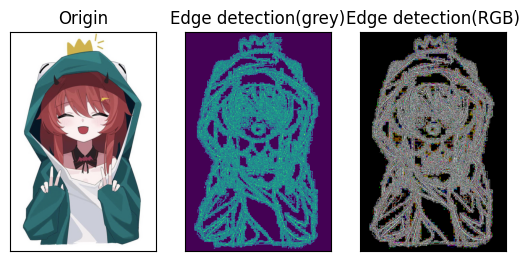$\left[\begin{array}{c} 0 & -1 & 0 \\ -1 & 5 & -1 \\ 0 & -1 & 0 \end{array}\right]$$\frac{1}{16}\left[\begin{array}{c} 1 & 2 & 1 \\ 2 & 4 & 2 \\ 1 & 2 & 1 \end{array}\right]$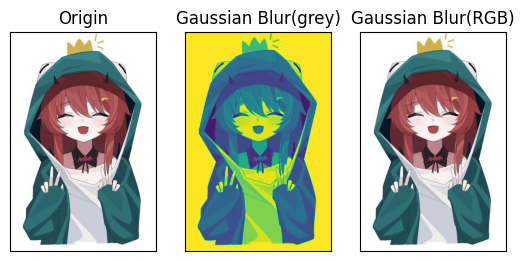#### 9. 交叉相关 vs 卷积

• 二维交叉相关
• 二维卷积
• 由于对称性，在实际使用中没有区别

#### 10. 一维和三维交叉相关

• 一维：文本、语言、时需序列
• 二维：视频、医学图像、气象图像

#### 11. 总结

• 卷积层将输入和核矩阵进行交叉相关，加上偏移后得到输出
• 核矩阵和偏移是可学习的参数
• 核矩阵的大小是超参数

### 四、LeNet

LeNet is a convolutional neural network structure proposed by LeCun et al. in 1998.

MNIST 是 LeNet 附带的数据集

• 5w个训练数据
• 1w个测试数据
• 图像大小$28 \times 28$
• 10类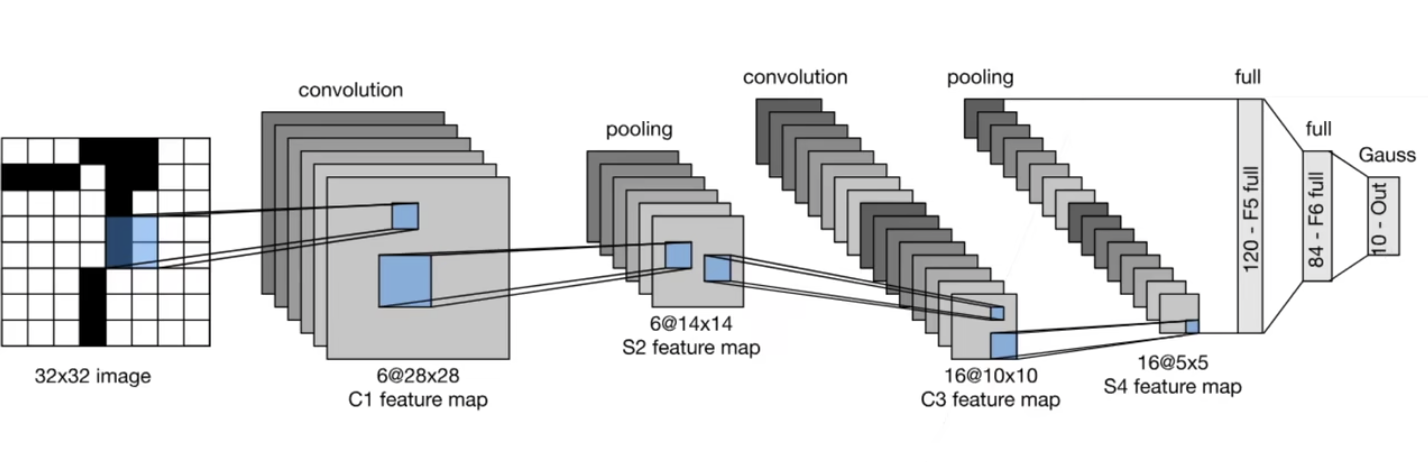#### 1. 小结

• LeNet是早期成功的神经网络
• 先使用卷积层来学习图片空间信息
• 然后使用全连接层来转换到类别空间

#### 2. 卷积神经网络（LeNet）

LeNet (LeNet-5) 由两个部分组成：卷积编码器和全连接层密集块

### 五、AlexNet

#### 1. 技术回顾

1）支持向量机

the Support Vector Machine (SVM)

• 特征提取
• 选择核函数来计算相似性
• 凸优化问题
• 漂亮的定理（来自泛函）

2）几何学

• 抽取特征
• 描述几何（例如多相机）
• （非）凸优化
• 漂亮定理
• 如果假设满足，效果很好

3）特征工程

• （在计算机视觉里）特征工程是关键
• 特征描述子：SIFT, SURF

#### 2. ImageNet (2020)

• 图片：自然物体的彩色图片
• 大小：$469 \times 387$
• 样本数 1.2M
• 类数：1000

#### 3. AlexNet

• AlexNet赢了2012年ImageNet竞赛
• 更深更大的 LeNet
• 主要改进：
• 丢弃法（因为模型大了，所以用丢弃做一点正则）
• ReLU（$Sigmoid \space (LeNet) \to ReLU$，ReLU在零点的一阶导更好，梯度更大）
• MaxPooling（数值比较大，梯度比较大，使得训练比较容易）
• 计算机视觉方法论的改变
• 核方法：人工特征提取 $\to$ SVM
• 神经网络：通过 CNN 学习特征 $\to$ Softmax回归

#### 4. AlexNet架构

1）第一个卷积层和池化层的区别

AlexNet LeNet
image ($3 \times 224 \times 224$) image ($32 \times 32$)
11*11 Conv(96), stride 4 5*5 Conv(6), pad 2
3*3 MaxPool, stride 2 2*2 AvgPool, stride 2
• 卷积：更大的窗口，更大的通道数，更大的步幅
• 池化：更大的窗口且stride不变（对位置更不敏感），使用MaxPool

2）接下来

AlexNet LeNet
5*5 Conv(256), pad 2 5*5 Conv()
3*3 MaxPool, stride 2 2*2 AvgPool, stride 2
3*3 MaxPool, stride 2
• 新增了3层卷积层
• 更多的输出通道

3）全连接层

AlexNet LeNet
Dense(4096) Dense(120)
Dense(4096) Dense(84)
Dense(1000) Dense(10)
• 隐藏层大小：120 $\to$ 4096
• 输出类别：10 $\to$ 1000

#### 5. 更多细节

• 激活函数从 Sigmoid 变到了 ReLU（减缓梯度消失，因为梯度比较大）
• 隐藏全连接层后加入了丢弃层
• 数据增强（随机截取采样，调亮度/色温。神经网络会记住所有数据，数据增强能让记住数据的能力变低）

#### 6. 总结

• AlexNet是更大更深的LeNet，10x参数个数，260x计算复杂度
• 新加入了丢弃法，ReLU，最大池化层，和数据增强
• AlexNet赢下了2012ImageNet竞赛后，标志着新的一轮神经网络热潮的开始

todo: 未完待续